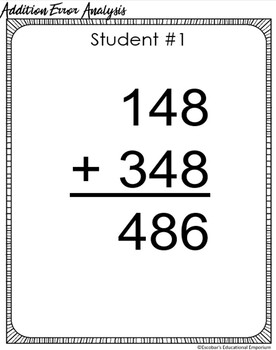Subject
Resource Type
File Type

PDF

(644 KB|17 pages)
Product Rating
Standards
• Product Description
• StandardsNEW

Included are 10 student examples where subtraction errors were made (errors with regrouping, not regrouping, subtracting instead of adding, etc). All error cards are done with the standard algorithm. Students must solve the problems correctly, then explain the error the other student made. One universal answer document is included with 10 answer keys.

Look for and express regularity in repeated reasoning. Mathematically proficient students notice if calculations are repeated, and look both for general methods and for shortcuts. Upper elementary students might notice when dividing 25 by 11 that they are repeating the same calculations over and over again, and conclude they have a repeating decimal. By paying attention to the calculation of slope as they repeatedly check whether points are on the line through (1, 2) with slope 3, middle school students might abstract the equation (𝑦 – 2)/(𝑥 – 1) = 3. Noticing the regularity in the way terms cancel when expanding (𝑥 – 1)(𝑥 + 1), (𝑥 – 1)(𝑥² + 𝑥 + 1), and (𝑥 – 1)(𝑥³ + 𝑥² + 𝑥 + 1) might lead them to the general formula for the sum of a geometric series. As they work to solve a problem, mathematically proficient students maintain oversight of the process, while attending to the details. They continually evaluate the reasonableness of their intermediate results.
Attend to precision. Mathematically proficient students try to communicate precisely to others. They try to use clear definitions in discussion with others and in their own reasoning. They state the meaning of the symbols they choose, including using the equal sign consistently and appropriately. They are careful about specifying units of measure, and labeling axes to clarify the correspondence with quantities in a problem. They calculate accurately and efficiently, express numerical answers with a degree of precision appropriate for the problem context. In the elementary grades, students give carefully formulated explanations to each other. By the time they reach high school they have learned to examine claims and make explicit use of definitions.
Fluently add and subtract multi-digit whole numbers using the standard algorithm.
Fluently add and subtract within 1000 using strategies and algorithms based on place value, properties of operations, and/or the relationship between addition and subtraction.
Total Pages
17 pages
Included
Teaching Duration
N/A
Report this Resource to TpT
Reported resources will be reviewed by our team. Report this resource to let us know if this resource violates TpT’s content guidelines.